### Home > CCAA8 > Chapter 8 Unit 9 > Lesson CCA: 8.1.2 > Problem8-17

8-17.

Use the process you developed in problem 8-14 to factor the following quadratics, if possible.

1. $x^2-4x-12$

Use a generic rectangle and a diamond to factor the quadratic.  Blank Diamond Problem. Draw a rectangle and divide it into four equal parts.

Added to the diamond problem: Left blank, Right blank, Top negative 12 x squared, Bottom negative 4 x. Added to the rectangle: Interior upper right box is negative 12. Interior lower left box is X squared.

Added to the diamond problem: Left negative 6 x, Right 2 x. Added to the rectangle: Interior upper left box is negative 6, X. Interior lower right box is 2, X.

Added to the rectangle exterior: The left edge top is negative 6. The left edge bottom is X. The bottom edge left is X. The bottom edge right is 2.$(x−6)(x+2)$

1. $4x^2+4x+1$

See the hints in part (a). Blank Diamond Problem. Draw a rectangle and divide it into four equal parts.

Added to the diamond problem: Left blank, Right blank, Top 4, x squared, Bottom 4, x. Added to the rectangle: Interior upper right box is 1. Interior lower left box is 4, X squared.

Added to the diamond problem: Left 2, x, Right 2, x. Added to the rectangle: Interior upper left box is 2, X. Interior lower right box is 2, X.

Added to the rectangle exterior: The left edge top is 1. The left edge bottom is 2, X. The bottom edge left is 2, X. The bottom edge right is 1.

$(2x+1)^2$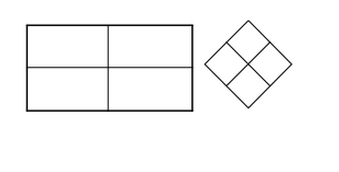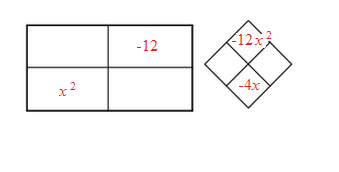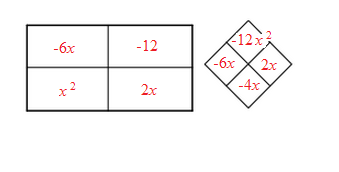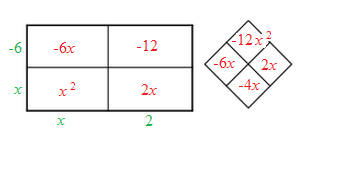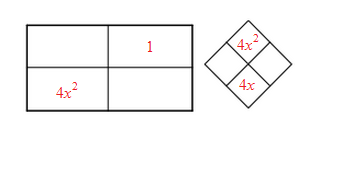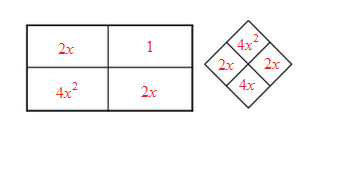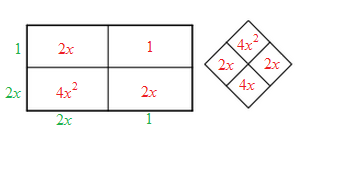1. $2x^2-9x-5$

See the hints in part (a). Blank Diamond Problem. Draw a rectangle and divide it into four equal parts.

Added to the diamond problem: Left blank, Right blank, Top negative 10, x squared, Bottom negative 9, x. Added to the rectangle: Interior upper right box is negative 5. Interior lower left box is 2, X squared.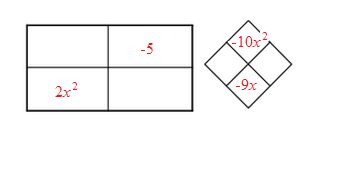1. $3x^2+10x-8$